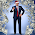Thursday, October 26, 2017

Magnetic Moment of the Proton, Final, The Real Case #3

$\textbf{CASE 3: Rotating Sphere of Uniform Surface-Charge}$
$$\bar{M}={4\pi\over3}\sigma r_p^4\bar{\omega}$$
from http://www-personal.umich.edu/~pran/jackson/P505/F07_hw08a.pdf

$\sigma=$ surface charge density
$a=$ radius of sphere, using proton radius, $r_p$
$\bar{\omega}=$ angular velocity(speed)
$e=$ elementary charge
$\sigma={e\over A_{sphere}}$
$A_{sphere}=4\pi r_p^2$
$\bar{\omega}={\triangle\Theta\over\triangle t}={2\pi\over{2\pi r_p\over c}}={c\over r_p}$
$\bar{M}={4\pi\over3}{e\over4\pi r_p^2}r_p^4{c\over r_p}$
$\bar{M}={er_pc\over3}$
$m_pr_p={2h\over\pi c}$
$r_p={2h\over\pi cm_p}$
$\hbar={h\over2\pi}$
$\bar{M}={e2hc\over3\pi cm_p}={2eh\over3\pi m_p}={2e2\pi\hbar\over3\pi m_p}={8e\hbar\over3\times2m_p}$
$\mu_N={e\hbar\over2m_p}$
$\bar{M}={8\over3}\mu_N$
$\bar{M}=2.666\mu_N$

$\mu_p=2.666\mu_N$
QED.

Now, what is this quark model for spin all about?

My proton magnetic moment is 4.5% less than the measured.  Does this sound like the familiar proton radius puzzle error?

Using CODATA value for proton radius and $\bar{M}={er_pc\over3}$, we find:
$\bar{M}={er_pc\over3}=1.40119247\times10^{-26}\,m^2 A$
(this is within 0.7% of CODATA value for proton magnetic moment)
(This, significantly, verifies the equation)

CODATA link for proton magnetic moment
($\mu_p=1.410 606 7873(97)\times10^{-26}\,J\,T^-1$)
(Google Calculated: using measured coeficient and fundamental constants

However, the new accurate value for the proton's magnetic moment is very likely:
($1.34687565\times10^{-26}\,m^2\,A$ <~~ This is about 4.5% less than present CODATA value)

https://en.wikipedia.org/wiki/Proton_spin_crisis - solved

Should see a Larmor frequency of about 40.65MHz/Tesla (hydrogen - $H^{+1}$):
(close to 40.77MHz and this FCC Band: ITU # 8 - VFH Very high frequency – FM Radio, TV broadcasts, and aircraft communications (30-300 MHz))
(when calibrated against a proton radius of $0.8412fm$ instead of $0.8751fm$)

http://bio.groups.et.byu.net/LarmourFreqCal.phtml

The Surfer, OM-IV
1.2.3.